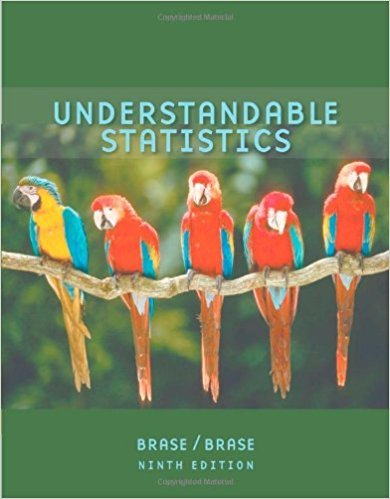×
×

# Solutions for Chapter 5: The Binomial Probability Distribution and Related Topics## Full solutions for Understandable Statistics | 9th Edition

ISBN: 9780618949922Solutions for Chapter 5: The Binomial Probability Distribution and Related Topics

Solutions for Chapter 5
4 5 0 416 Reviews
11
4
##### ISBN: 9780618949922

This expansive textbook survival guide covers the following chapters and their solutions. Chapter 5: The Binomial Probability Distribution and Related Topics includes 20 full step-by-step solutions. Since 20 problems in chapter 5: The Binomial Probability Distribution and Related Topics have been answered, more than 38322 students have viewed full step-by-step solutions from this chapter. This textbook survival guide was created for the textbook: Understandable Statistics, edition: 9. Understandable Statistics was written by and is associated to the ISBN: 9780618949922.

Key Statistics Terms and definitions covered in this textbook
• 2 k factorial experiment.

A full factorial experiment with k factors and all factors tested at only two levels (settings) each.

• Additivity property of x 2

If two independent random variables X1 and X2 are distributed as chi-square with v1 and v2 degrees of freedom, respectively, Y = + X X 1 2 is a chi-square random variable with u = + v v 1 2 degrees of freedom. This generalizes to any number of independent chi-square random variables.

• Alternative hypothesis

In statistical hypothesis testing, this is a hypothesis other than the one that is being tested. The alternative hypothesis contains feasible conditions, whereas the null hypothesis speciies conditions that are under test

• Bimodal distribution.

A distribution with two modes

• Chi-square (or chi-squared) random variable

A continuous random variable that results from the sum of squares of independent standard normal random variables. It is a special case of a gamma random variable.

• Combination.

A subset selected without replacement from a set used to determine the number of outcomes in events and sample spaces.

• Comparative experiment

An experiment in which the treatments (experimental conditions) that are to be studied are included in the experiment. The data from the experiment are used to evaluate the treatments.

• Conditional probability

The probability of an event given that the random experiment produces an outcome in another event.

• Conidence coeficient

The probability 1?a associated with a conidence interval expressing the probability that the stated interval will contain the true parameter value.

• Contingency table.

A tabular arrangement expressing the assignment of members of a data set according to two or more categories or classiication criteria

• Correction factor

A term used for the quantity ( / )( ) 1 1 2 n xi i n ? = that is subtracted from xi i n 2 ? =1 to give the corrected sum of squares deined as (/ ) ( ) 1 1 2 n xx i x i n ? = i ? . The correction factor can also be written as nx 2 .

• Critical region

In hypothesis testing, this is the portion of the sample space of a test statistic that will lead to rejection of the null hypothesis.

• Crossed factors

Another name for factors that are arranged in a factorial experiment.

• Degrees of freedom.

The number of independent comparisons that can be made among the elements of a sample. The term is analogous to the number of degrees of freedom for an object in a dynamic system, which is the number of independent coordinates required to determine the motion of the object.

• Deming

W. Edwards Deming (1900–1993) was a leader in the use of statistical quality control.

• Erlang random variable

A continuous random variable that is the sum of a ixed number of independent, exponential random variables.

• Error variance

The variance of an error term or component in a model.

• First-order model

A model that contains only irstorder terms. For example, the irst-order response surface model in two variables is y xx = + ?? ? ? 0 11 2 2 + + . A irst-order model is also called a main effects model

• Generating function

A function that is used to determine properties of the probability distribution of a random variable. See Moment-generating function

• Goodness of fit

In general, the agreement of a set of observed values and a set of theoretical values that depend on some hypothesis. The term is often used in itting a theoretical distribution to a set of observations.

×